# Cartographic Projections

The following article is from The Great Soviet Encyclopedia (1979). It might be outdated or ideologically biased.

## Cartographic Projections

representations of the entire surface of the earth’s ellipsoid or some part of it on a plane; produced mainly for the construction of a map.

Scale. Cartographic projections are made on a definite scale. By hypothetically reducing the earth’s ellipsoid by a factor of M— for example, 10, 000, 000—we obtain a geometric model of it, a globe, the representation of which on a plane in actual size gives a map of the surfaces of the ellipsoid. The quantity 1 \M (in this case, 1:10, 000, 000) defines the principal, or general, scale of the map. Because the surfaces of the ellipsoid and sphere cannot be shown on a plane without breaks and folds (they do not belong to the class of developable surfaces), any cartographic projection inherently contains distortions of the length of lines and of angles typical of any map. The basic characteristic of any cartographic projection at any point on it is the particular scale μ. This is a quantity that is the inverse of the ratio of an infinitely small segment ds on the earth’s ellipsoid to its representation do- on the plane: 1/μ, = ds/dσ, where ja depends on the position of the point on the ellipsoid and on the direction of the segment selected. It is clear that μ, min ≤μ, ≤μmax and that equality is possible here only at individual points or along certain lines on the map. Thus, the principal scale of the map describes it only in general, in a certain average sense. The ratio μ/M is called the relative scale or the magnification of a line, and the difference μ/M — 1 is called the distortion of the line. In the analysis of the characteristics of a cartographic projection, the principal scale may be neglected; its numerical value is considered only during computation of the coordinates of points of the cartographic projection. Therefore, M is often taken as equal to 1 (for example, in the theory of distortions).

General information. The purpose of the theory of cartographic projections (mathematical cartography) is the study of all types of distortions of plane representations of the surface of the earth’s ellipsoid and the development of methods for constructing projections in which distortions have a minimum value (in some sense) or a predetermined distribution.

Proceeding from the needs of cartography, the theory of cartographic projections deals with representations of the surface of the earth’s ellipsoid on a plane. Since the earth’s ellipsoid is slightly compressed and its surface deviates slightly from that of a sphere, and also because cartographic projections are needed to draw maps in medium and small scales (M > 1,000, 000), consideration is often limited to representations on a plane of a sphere with radius R whose deviations from the ellipsoid can be neglected or somehow taken into account. Therefore, we then have in mind representations on the plane xOy of a sphere relative to the geographic coordinates ϕ(latitude) and λ (longitude).

The equations of any cartographic projection have the form

(1) x =f1(ϕ, λ) y = f2(ϕ, λ)

where f1 and f2 are functions that satisfy certain general conditions. The representations of the meridians λ = const, and the parallels ϕ = const, in the given cartographic projection form the cartographic grid. The projection may also be defined by two equations that use some coordinates other than the rectangular x and y coordinates of the plane. Some projections—for example, perspective projections (in particular, orthographic projections; see Figure 2) and perspective-cylindrical projections (Figure 7)—may be defined by geometric constructions. A cartographic projection is also defined by the rule of construction of a corresponding cartographic grid or by such characteristic properties as can be used to obtain equations of the form of (1) that fully define the projection.

Brief historical survey. The development of the theory of cartographic projections, like that of cartography as a whole, is closely associated with the development of geodesy, geography, astronomy, and mathematics.

The scientific foundations of cartography were laid in ancient Greece (sixth to first centuries b.C.). The gnomonic projection used by Thales in making star maps is considered to be the oldest cartographic projection. The development and use of cartographic projections in the compilation of geographic maps (by Hipparchus, Ptolemy, and others) began after the earth was found to be spheroidal (third century b.C.).

The significant upsurge of cartography in the 16th century brought about by the great geographical discoveries led to the creation of a number of new projections; one of them, proposed by G. Mercator, is still used today. In the 17th and 18th centuries, when the widespread organization of topographic surveying began to provide reliable material for the compilation of maps of significant territories, cartographic projections were developed as the basis for topographic maps (the French cartographer R. Bonne; G. D. Cassini) and the most important groups of cartographic projections were studied (J. Lambert, L. Euler, and J. Lagrange).

The development of military cartography and a further increase in the scope of topographic work in the 19th century required a secure mathematical foundation for large-scale maps and the institution of a system of retangular coordinates based on a more suitable cartographic projection. This requirement led to the development by K. Gauss of the fundamental geodetic projection. Finally, in the mid-19th century A. Tissot (France) produced a general theory of distortions of cartographic projections.

The development of the theory of cartographic projections in Russia was closely tied to practical needs and produced many original results (L. Euler, F. I. Shubert, P. L. Chebyshev, and D. A. Grave). New groups of cartographic projections and specific variations of them (to the stage of practical use) were developed and important questions of the general theory of projections and their classification were treated in the works of Soviet cartographers V. V. Kavraiskii and N. A. Urmaev.

Theory of distortions. Distortions in the infinitely small area around any point of a cartographic projection follow certain general rules. At any point on a map in a projection that is not conformal, there are two perpendicular directions that correspond to two perpendicular directions on the surface being represented; these are the cardinal directions of representation. The scales in these directions (cardinal scales) have extreme values: μmax = a and μmin = b. If the meridians and parallels on a map in any projection intersect at right angles, their directions are the cardinal directions for that projection. The distortion of length at the given point of the projection graphically represents the ellipse of distortion, which resembles (and is located similarly to) the representation of an infinitely small circle inscribed around the corresponding point of the surface being depicted. The radii of the ellipse are numerically equal to the particular scales at the given point in the corresponding directions; the semiaxes of the ellipse are equal to the extreme scales, and their directions are cardinal.

The relationship among elements of the ellipse of distortion, the distortions of the cartographic projection, and the partial derivatives of functions (1) is established by the basic formulas of the theory of distortions.

Classification according to position of the pole of the spherical coordinates. The poles of a sphere are special points of geographic coordination, although the sphere does not have any special characteristics at these points. Thus, when mapping areas that have geographic poles, the use of coordinates in which the poles are ordinary points of coordination, rather than geographic coordinates, is sometimes desirable. Therefore, spherical coordinates whose coordinate lines—the verticals (on which the conventional longitude a = const.) and altitude circles (almu-cantars), where the polar distances z = const.—are similar to the geographic meridians and parallels, except that their pole Z0 does not coincide with the geographic pole P0(Figure 1), are used on a sphere. The conversion from the geographic coordinates ϕ and λ of any point on a sphere to its spherical coordinates z and a for a given position of the pole Z0o »o) is carried out according to the formulas of spherical trigonometry. Any cartographic projection given by equations (1) is called normal (ϕ0 = π/2). If the same projection of a sphere is computed according to the same formulas (1) but with z and a in place of and A, the projection is called transverse for 0 = 0 and oblique for 0 < ϕ0 < π/2. The use of oblique and transverse projections reduces distortion. Normal (A), transverse (B), and oblique (C) orthographic projections of a sphere (surface of a globe) are shown in Figure 2.

Classification according to nature of distortions. In conformal cartographic projections the scale depends only on the position of a point, not on its direction. The ellipses of distortion degenerate into circles. Examples are the Mercator and stereographic projections.

In equal-area (equivalent) projections, areas are preserved; more precisely, the areas of figures on maps made in such projections are proportional to the areas of the corresponding actual figures, and the proportionality constant is inverse to the square of the principal scale of the map. The ellipses of distortion always have the same area but differ in shape and orientation.

Conventional cartographic projections are neither conformal nor equal-area. The most important projections of this type are the equidistant projections, in which one of the principal scales is equal to 1, and the orthodromic projections, in which the great circles (orthodromes) are represented by straight lines.

When a sphere is represented on a plane the features of confor-mality, equal area, equal distance, and orthodromes are incompatible. The following are used to show distortions at different places in the area represented: (1) ellipses of distortion constructed in various places on the grid or sketch of the map (Figure 3); (2) isokols, or lines of equal distortion value (in Figure 8, C, see the isokols of greatest distortion of angles co and the isokols of the scale of areas P); (3) the representation of certain spherical lines, usually orthodromes (O) and loxodromes (L ), at certain places on the map (see Figures 3, A and 3, B).

Classification of normal projections according to type of representation of meridians and parallels. Classification according to type of representation of meridians and parallels results from the historical development of the theory of cartographic projections and covers most known projections. It has preserved the names related to the geometric method of producing projections, but the groups considered are now defined analytically.

The cylindrical projections (Figure 3) are projections in which the meridians are shown by equidistant parallel straight lines and the parallels are straight lines perpendicular to the meridians. Such projections are advantageous for showing territories that extend along the equator or a parallel. The Mercator projection, a conformal cylindrical projection, is used in navigation. The Gauss-Krueger projection, a conformal transverse cylindrical projection, is used in the compilation of topographic maps and in triangulation.

The conic projections (Figure 4) are projections in which the parallels are shown by concentric circles and the meridians by straight lines orthogonal to them. In these projections distortion does not depend on longitude. They are especially suitable for territories that extend along parallels. Maps of the entire territory of the USSR are often made in conic orthomorphic and conic equidistant projections. They are also used as geodetic projections.

Azimuthal projections (Figure 5) are projections in which the parallels are concentric circles and the meridians are their radii, with the angles between the meridians equal to the corresponding differences in longitude. Perspective projections are a particular case of azimuthal projections.

Pseudoconic projections (Figure 6) are projections in which the parallels are represented by concentric circles, the central meridian is a straight line, and the other meridians are curves. The Bonne equal-area pseudoconic projection is common; it was used for a 3-verst (1:126, 000) map of the European part of Russia in 1847 (1 verst = 1.07 km).

Pseudocylindrical projections (Figure 8) are projections in which the parallels are represented by parallel straight lines and the central meridian is a straight line perpendicular to these straight lines and serves as the axis of symmetry of the projections, whereas the other meridians are curves.

In polyconic projections (Figure 9), the parallels are represented by circles whose centers are located on one straight line that represents the central meridian. Additional conditions are imposed in the construction of any given polyconic projections. One of the polyconic projections has been recommended for the international (1:1,000, 000) map.

There are many projections that are not classified with the types mentioned above. Cylindrical, conic, and azimuthal projections, called simple projections, are often classified as circular projections in the broad sense, separating the circular projections in the narrow sense (projections in which all meridians and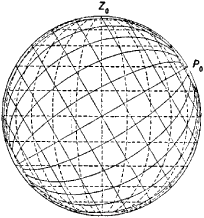Figure 1. Grids of spherical coordinate lines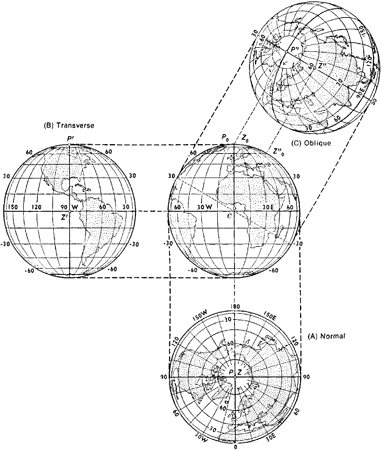Figure 2. The globe and its orthographic projections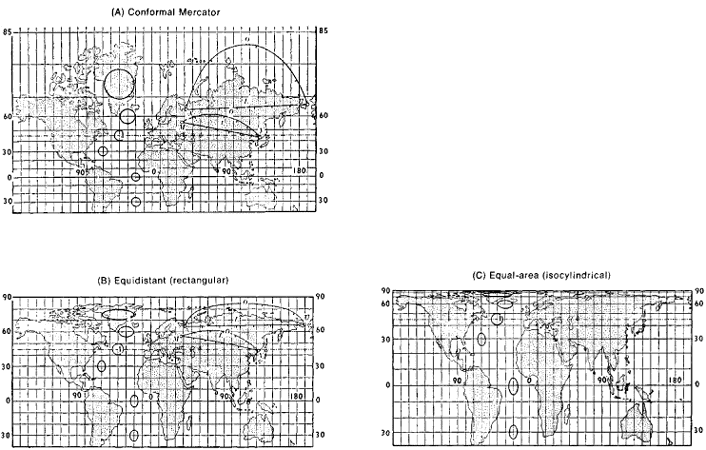Figure 3. Cylindrical projections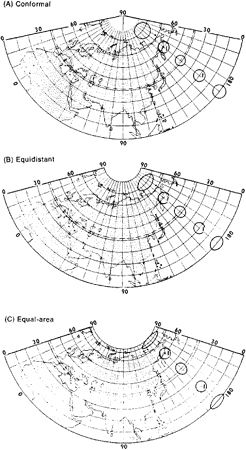Figure 4. Conic projections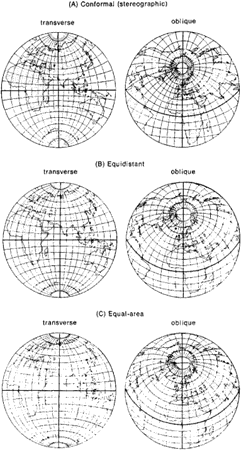Figure 5. Azimuthal projections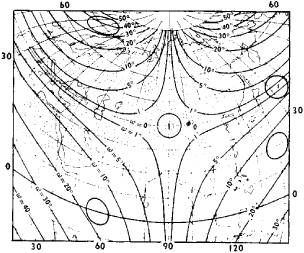Figure 6. Bonne pseudoconic equal-area projection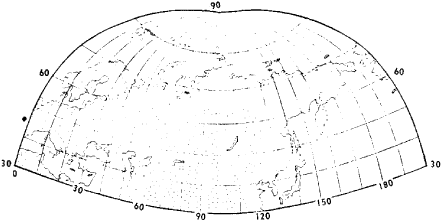Figure 7. Oblique perspective-cylindrical projection of M. D. Solov’ev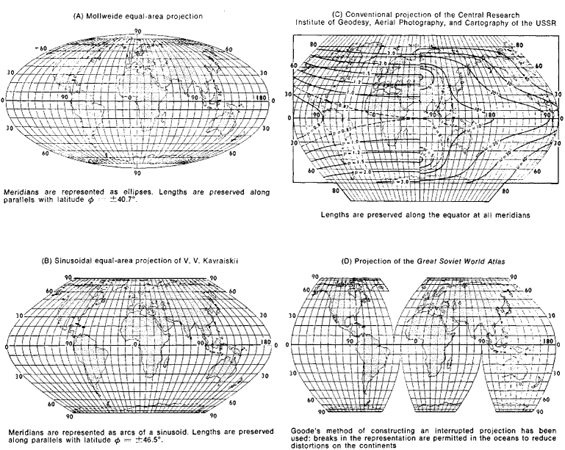Figure 8. Pseudocylindrical projections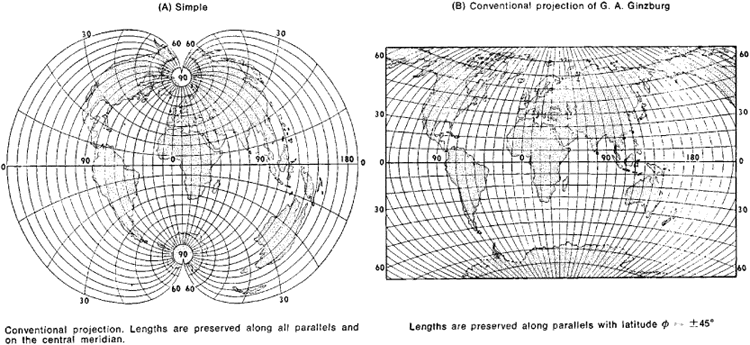Figure 9. Polyconic projections

parallels are represented by circles—for example, the conformal Lagrange projections and the Grinten projection).

Use and selection. The use and selection of cartographic projections depend primarily on the purpose and scale of the map, which determine the nature of permissible distortions in the cartographic projection chosen. Large-scale and medium-scale maps intended for measurement are usually made in conformal projections; small-scale maps to be used for general surveys and to determine the ratio of the areas of certain territories are made in equal-area projections. In this case there may be some violation of the determining conditions of the projections (ω \$ 0 or p \$ 1) without causing tangible error; in other words, the selection of conventional projections is permissible. Projections with equal intervals along the meridians are most often used in this case, as well as when the purpose of the map does not entail any preservation of angles or areas. When selecting a cartographic projection, one begins with the simplest types and then proceeds to the more complex projections, possibly even modifying them. If none of the known cartographic projections meets the requirements made of the map being drawn in relation to its purpose, then a new, most appropriate cartographic projection is sought. Distortion is minimized. The problem of constructing optimal projections, in which all types of distortions have been reduced to a minimum, has not yet been fully resolved.

Cartographic projections are also used in navigation, astronomy, and crystallography. Projections for mapping the moon, the planets, and other celestial bodies are being sought.

Conversion. When considering two cartographic projections given by the corresponding systems of equations x = f1(ϕ, λ), y = f2(ϕ, λ) and X = g1(ϕ, λ), Y = g2(ϕ, λ), one may establish the conversion from one projection to the other by removing ϕ and λ from these equations:

X = F1((x, y) Y = F2(x, y)

When the type of functions F1 and F2 is concretely determined, these formulas give a general method for obtaining the derivatives of the projections and make up the theoretical basis for all possible methods and technical procedures of map-making. For example, affine and linear fractional transformations are performed by means of cartographic converters. However, more general conversions require the use of new technology, particularly electronic equipment. The problem of building improved converters of cartographic projections is a pressing problem of modern cartography.

### REFERENCES

Vitkovskii, V. Kartografiia (Teoriia kartograficheskikh proektsii). St. Petersburg, 1907.
Kavraiskii, V. V. Matematicheskaia kartografiia. Moscow-Leningrad, 1934.
Kavraiskii, V. V. Izbr. trudy, vol. 2, issues 1–3. [Moscow] 1958–60.
Urmaev, N. A. Matematicheskaia kartografiia. Moscow, 1941.
Urmaev, N. A. Metody izyskaniia novykh kartograficheskikh proektsii. Moscow, 1947.
Graur, A. V. Matematicheskaia kartografiia, 2nd ed. Leningrad, 1956.
Ginzburg, G. A. Kartograficheskie proektsii. Moscow, 1951.
Meshcheriakov, G. A. Teoreticheskie osnovy matematicheskoi karto-grafii. Moscow, 1968.

G. A. MESHCHERIAKOV